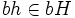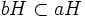# Left cosets of a subgroup partition a monoid

Jump to: navigation, search

## Statement

Suppose$G$ is a monoid and$H$ is a subgroup of$G$, i.e.,$H$ is a subset of$G$ with the same identity element and forming a group under the induced binary operation. Then, the following equivalent conditions hold:

1. The left cosets of$H$, namely$gH, g \in G$, form a partition of$G$. In other words,$G$ is a disjoint union of all the left cosets of$H$ in$G$.
2. The relation$a \sim b \iff a \in bH$ is an equivalence relation on$G$.
3. For every$g \in G$, there is exactly one left coset of$G$ containing$g$.
4. For$a,b \in G$, either$aH = bH$ or$aH \cap bH$ is empty.

## Equivalence of statements

These statements are equivalent because of the following general fact about sets and equivalence relations. If$S$ is a set, and$\sim$ is an equivalence relation on$S$, then we can partition$S$ as a disjoint union of equivalence classes under$\sim$. Two elements$a$ and$b$ are defined to be in the same equivalence class under$\sim$ if$a \sim b$.

Conversely, if$S$ is partitioned as a disjoint union of subsets, then the relation of being in the same subset is an equivalence relation on$S$.

Hence, there is a correspondence between equivalence relations on a set and partitions of the set into subsets. This statement about left cosets thus states that the left cosets partition the group, which is also the same as saying that the relation of one element being in the left coset of another, is an equivalence relation.

Here, we give the proof both in form (2) and form (4). The two proofs are essentially the same, but they are worked out in somewhat different language, and explain how to think both in terms of equivalence relations and in terms of partitions.

## Proof in form (2)

Given: A monoid$G$, a subgroup$H$

To prove: The relation$a \sim b \iff \ \exists \ h \in H$ such that$a = bh$, is an equivalence relation on$G$.

Note that in the proof, we will freely use the existence of inverses of elements in$H$ as well as associativity in$G$. Further, it is important for the proofs that the identity element of$H$ is the same as that of$G$.

### Reflexivity

To prove: For any$a \in G$,$a \sim a$.

Proof: Clearly$e \in H$ (since$H$ is a subgroup with the same identity element). Hence, for any$a \in G$,$a = ae$, so$a \sim a$:$a$ is in its own left coset.

### Symmetry

To prove: For any$a,b \in G$ such that$a \sim b$, we have$b \sim a$.

Proof: If$a = bh$, for some$h \in H$, then$ah^{-1} = (bh)h^{-1} = b(hh^{-1}) = b$. Since$h \in H$ and$H$ is a subgroup,$h^{-1} \in H$. Thus, if$a$ is in the left coset of$b$, then$b$ is in the left coset of$a$. In symbols,$a \sim b \implies b \sim a$.

### Transitivity

To prove: If$a,b,c \in G$ are such that$a \sim b$, and$b \sim c$, then$a \sim c$

Proof: If$a = bh$, and$b = ck$, for$h, k \in H$, and$a = (ck)h = c(kh)$. Since$H$ is a subgroup,$h,k \in H \implies kh \in H$, so$a$ is in the left coset of$c$.

## Proof in form (4)

Given: A monoid$G$, a subgroup$H$, two elements$a,b \in G$

To prove: The left cosets$aH$ and$bH$ are either equal or disjoint (they have empty intersection)

Proof: We'll assume that$aH$ and$bH$ are not disjoint, and prove that they are equal.

For this, suppose$c \in aH \cap bH$. Then, there exist$h_1,h_2$ such that$ah_1 = bh_2 = c$. Thus,$b = ah_1h_2^{-1} \in aH$ and$a = bh_2h_1^{-1} \in bH$.

Now, for any element$ah \in aH$, we have$ah = bh_2h_1^{-1}h \in bH$, and similarly, for every element$bh \in bH$, we have$bh = ah_1h_2^{-2}h \in aH$. Thus,$aH \subset bH$ and$bH \subset aH$, so$aH = bH$.

## Other proofs

### Orbits under a group action

One easy way of seeing that the left cosets partition a monoid is by viewing the left cosets as orbits of the monoid under the action of the subgroup by right multiplication.